HomeMachine LearningMachine Learning EducationDeep Learning Algorithms One Should Know

# Deep Learning Algorithms One Should Know

 Audio version of the article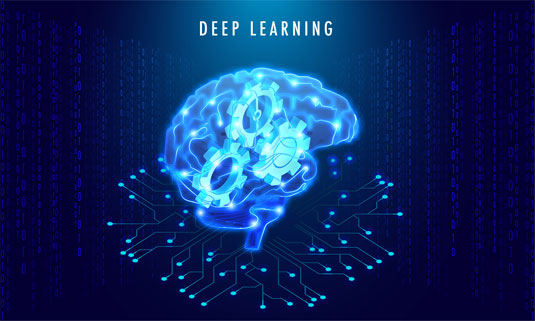Deep learning has gained massive popularity in scientific computing, and its algorithms are widely used by industries that solve complex problems. All deep learning algorithms use different types of neural networks to perform specific tasks.

This article examines essential artificial neural networks and how deep learning algorithms work to mimic the human brain. It covers the following topics

• What is Deep Learning?
• Defining Neural Networks
• How does Deep Learning algorithm work?
• Types of Deep Learning algorithms

## What is Deep Learning?

Deep learning uses artificial neural networks to perform sophisticated computations on large amounts of data. It is a type of machine learning that works based on the structure and function of the human brain.

Deep learning algorithms train machines by learning from examples. Industries such as health care, eCommerce, entertainment, and advertising commonly use deep learning.

## Defining Neural Networks

A neural network is structured like the human brain and consists of artificial neurons, also known as nodes. These nodes are stacked next to each other in three layers:

• The input layer
• The hidden layer(s)
• The output layer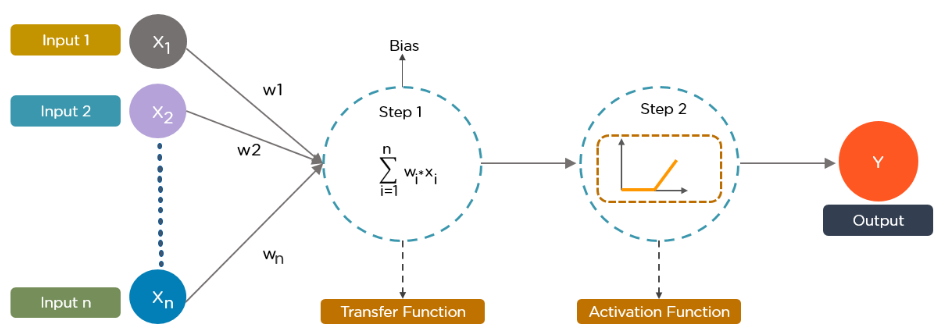Data provides each node with information in the form of inputs. The node multiplies the inputs with random weights, calculates them, and adds a bias. Finally, nonlinear functions, also known as activation functions, are applied to determine which neuron to fire.

## How Deep Learning Algorithms Work?

While deep learning algorithms feature self-learning representations, they depend upon ANNs that mirror the way the brain computes information. During the training process, algorithms use unknown elements in the input distribution to extract features, group objects, and discover useful data patterns. Much like training machines for self-learning, this occurs at multiple levels, using the algorithms to build the models.

Deep learning models make use of several algorithms. While no one network is considered perfect, some algorithms are better suited to perform specific tasks. To choose the right ones, it’s good to gain a solid understanding of all primary algorithms.

## Types of Deep Learning Algorithms

1. Convolutional Neural Networks (CNNs)
2. Long Short Term Memory Networks (LSTMs)
3. Recurrent Neural Networks (RNNs)
4. Generative Adversarial Networks (GANs)
5. Radial Basis Function Networks (RBFNs)
6. Multilayer Perceptrons (MLPs)
7. Self Organizing Maps (SOMs)
8. Deep Belief Networks (DBNs)
9. Restricted Boltzmann Machines( RBMs)
10. Autoencoders

Deep learning algorithms work with almost any kind of data and require large amounts of computing power and information to solve complicated issues. Now, let us, deep-dive, into the top 10 deep learning algorithms.

### 1. Convolutional Neural Networks (CNNs)

CNN’s, also known as ConvNets, consist of multiple layers and are mainly used for image processing and object detection. Yann LeCun developed the first CNN in 1988 when it was called LeNet. It was used for recognizing characters like ZIP codes and digits.

CNN’s are widely used to identify satellite images, process medical images, forecast time series, and detect anomalies.

#### How Do CNNs Work?

CNN’s have multiple layers that process and extract features from data:

#### Convolution Layer

• CNN has a convolution layer that has several filters to perform the convolution operation.

#### Rectified Linear Unit (ReLU)

• CNN’s have a ReLU layer to perform operations on elements. The output is a rectified feature map.

#### Pooling Layer

• The rectified feature map next feeds into a pooling layer. Pooling is a down-sampling operation that reduces the dimensions of the feature map.
• The pooling layer then converts the resulting two-dimensional arrays from the pooled feature map into a single, long, continuous, linear vector by flattening it.

Fully Connected Layer

• A fully connected layer forms when the flattened matrix from the pooling layer is fed as an input, which classifies and identifies the images.

Below is an example of an image processed via CNN.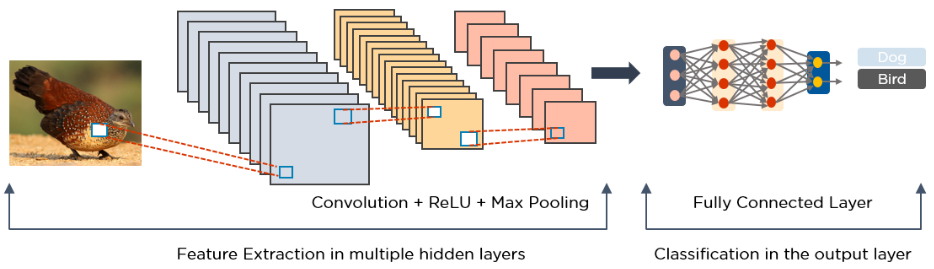### 2. Long Short Term Memory Networks (LSTMs)

LSTMs are a type of Recurrent Neural Network (RNN) that can learn and memorize long-term dependencies. Recalling past information for long periods is the default behavior.

LSTMs retain information over time. They are useful in time-series prediction because they remember previous inputs. LSTMs have a chain-like structure where four interacting layers communicate in a unique way. Besides time-series predictions, LSTMs are typically used for speech recognition, music composition, and pharmaceutical development.

#### How Do LSTMs Work?

• First, they forget irrelevant parts of the previous state
• Next, they selectively update the cell-state values
• Finally, the output of certain parts of the cell state

Below is a diagram of how LSTMs operate: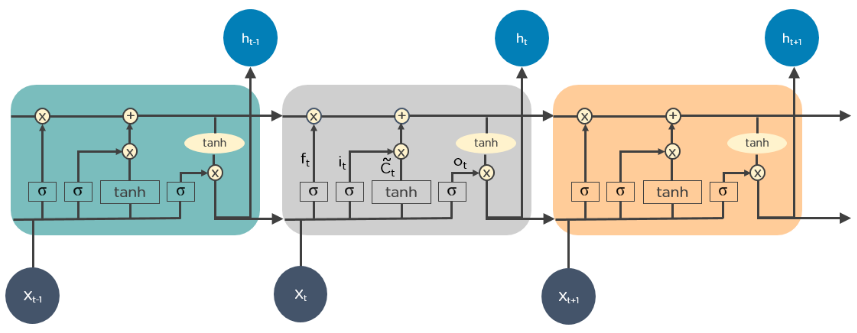### 3. Recurrent Neural Networks (RNNs)

RNNs have connections that form directed cycles, which allow the outputs from the LSTM to be fed as inputs to the current phase.

The output from the LSTM becomes an input to the current phase and can memorize previous inputs due to its internal memory. RNNs are commonly used for image captioning, time-series analysis, natural-language processing, handwriting recognition, and machine translation.

An unfolded RNN looks like this: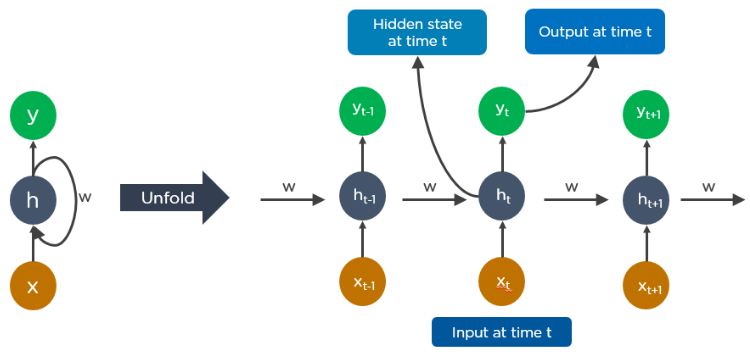#### How Do RNNs work?

• The output at time t-1 feeds into the input at time t.
• Similarly, the output at time t feeds into the input at time t+1.
• RNNs can process inputs of any length.
• The computation accounts for historical information, and the model size does not increase with the input size.

Here is an example of how Google’s autocompleting feature works: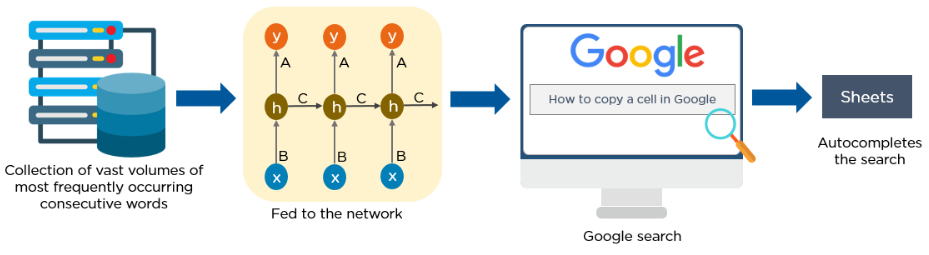### 4. Generative Adversarial Networks (GANs)

GANs are generative deep learning algorithms that create new data instances that resemble the training data. GANs have two components: a generator, which learns to generate fake data, and a discriminator, which learns from that false information.

The usage of GANs has increased over a period of time. They can be used to improve astronomical images and simulate gravitational lensing for dark-matter research. Video game developers use GANs to upscale low-resolution, 2D textures in old video games by recreating them in 4K or higher resolutions via image training.

GANs help generate realistic images and cartoon characters, create photographs of human faces, and render 3D objects.

#### How Do GANs work?

• The discriminator learns to distinguish between the generator’s fake data and the real sample data.
• During the initial training, the generator produces fake data, and the discriminator quickly learns to tell that it’s false.
• The GAN sends the results to the generator and the discriminator to update the model.

Below is a diagram of how GANs operate: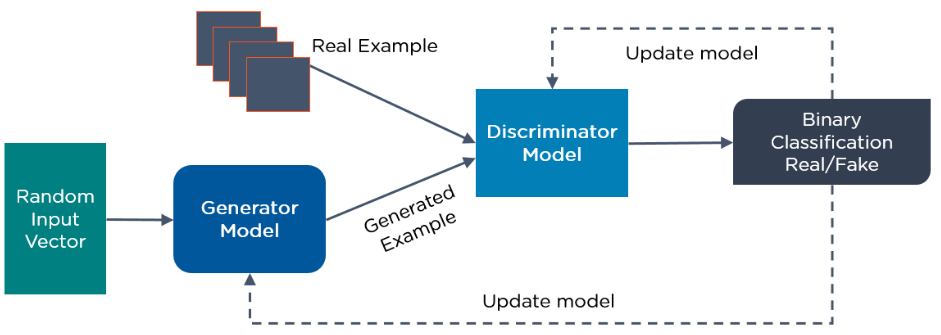### 5. Radial Basis Function Networks (RBFNs)

RBFNs are special types of feedforward neural networks that use radial basis functions as activation functions. They have an input layer, a hidden layer, and an output layer and are mostly used for classification, regression, and time-series prediction.

#### How Do RBFNs Work?

• RBFNs perform classification by measuring the input’s similarity to examples from the training set.
• RBFNs have an input vector that feeds to the input layer. They have a layer of RBF neurons.
• The function finds the weighted sum of the inputs, and the output layer has one node per category or class of data.
• The neurons in the hidden layer contain the Gaussian transfer functions, which have outputs that are inversely proportional to the distance from the neuron’s center.
• The network’s output is a linear combination of the input’s radial-basis functions and the neuron’s parameters.

See this example of an RBFN: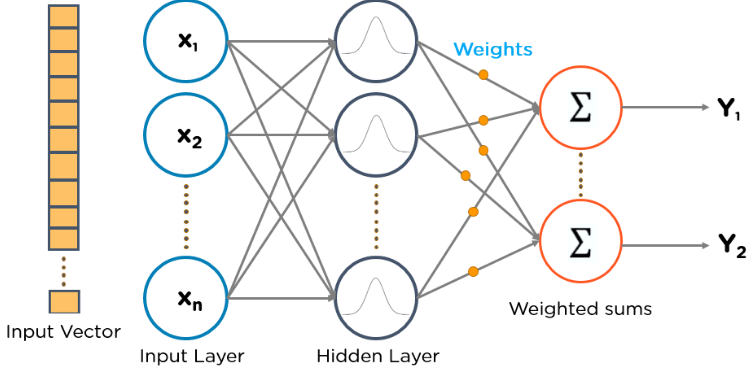### 6. Multilayer Perceptrons (MLPs)

MLPs are an excellent place to start learning about deep learning technology.

MLPs belong to the class of feedforward neural networks with multiple layers of perceptrons that have activation functions. MLPs consist of an input layer and an output layer that are fully connected. They have the same number of input and output layers but may have multiple hidden layers and can be used to build speech-recognition, image-recognition, and machine-translation software.

#### How Do MLPs Work?

• MLPs feed the data to the input layer of the network. The layers of neurons connect in a graph so that the signal passes in one direction.
• MLPs compute the input with the weights that exist between the input layer and the hidden layers.
• MLPs use activation functions to determine which nodes to fire. Activation functions include ReLUs, sigmoid functions, and tanhs.
• MLPs train the model to understand the correlation and learn the dependencies between the independent and the target variables from a training data set.

Below is an example of an MLP. The diagram computes weights and bias and applies suitable activation functions to classify images of cats and dogs.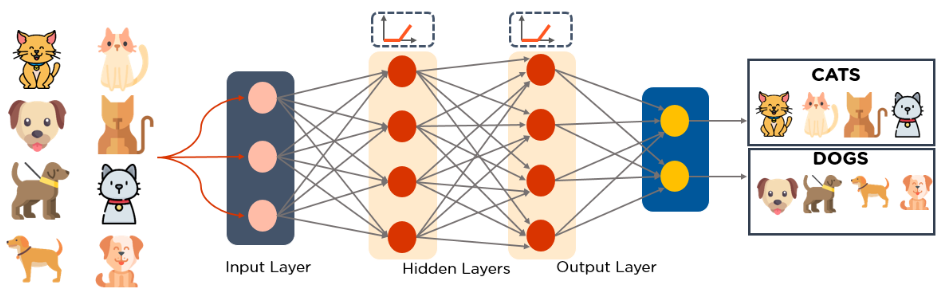### 7. Self Organizing Maps (SOMs)

Professor Teuvo Kohonen invented SOMs, which enable data visualization to reduce the dimensions of data through self-organizing artificial neural networks.

Data visualization attempts to solve the problem that humans cannot easily visualize high-dimensional data. SOMs are created to help users understand this high-dimensional information.

#### How Do SOMs Work?

• SOMs initialize weights for each node and choose a vector at random from the training data.
• SOMs examine every node to find which weights are the most likely input vector. The winning node is called the Best Matching Unit  (BMU).
• SOMs discover the  BMU’s neighborhood, and the amount of neighbors lessens over time.
• SOMs award a winning weight to the sample vector. The closer a node is to a BMU, the more its weight changes..
• The further the neighbor is from the BMU, the less it learns. SOMs repeat step two for N iterations.

Below, see a diagram of an input vector of different colors. This data feeds to a SOM, which then converts the data into 2D RGB values. Finally, it separates and categorizes the different colors.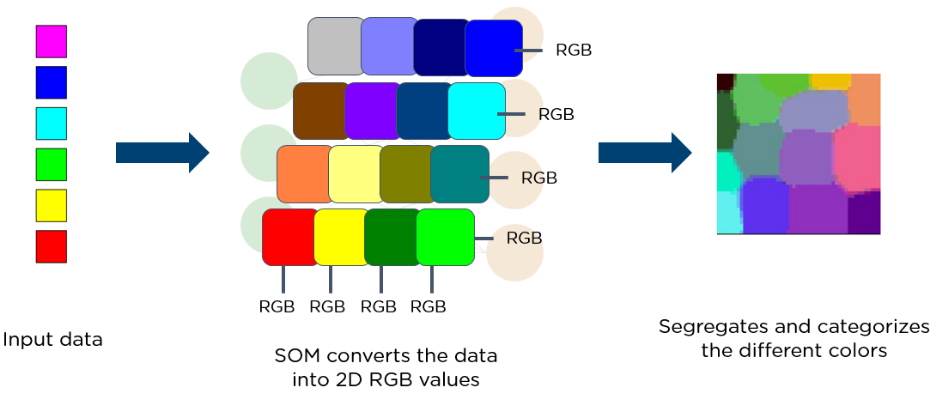### 8. Deep Belief Networks (DBNs)

DBNs are generative models that consist of multiple layers of stochastic, latent variables. The latent variables have binary values and are often called hidden units.

DBNs are a stack of Boltzmann Machines with connections between the layers, and each RBM layer communicates with both the previous and subsequent layers. DBNs are used for image-recognition, video-recognition, and motion-capture data.

#### How Do DBNs Work?

• Greedy learning algorithms train DBNs. The greedy learning algorithm uses a layer-by-layer approach for learning the top-down, generative weights.
• DBNs run the steps of Gibbs sampling on the top two hidden layers. This stage draws a sample from the RBM deﬁned by the top two hidden layers.
• DBNs draw a sample from the visible units using a single pass of ancestral sampling through the rest of the model.
• DBNs learn that the values of the latent variables in every layer can be inferred by a single, bottom-up pass.

Below is an example of DBN architecture: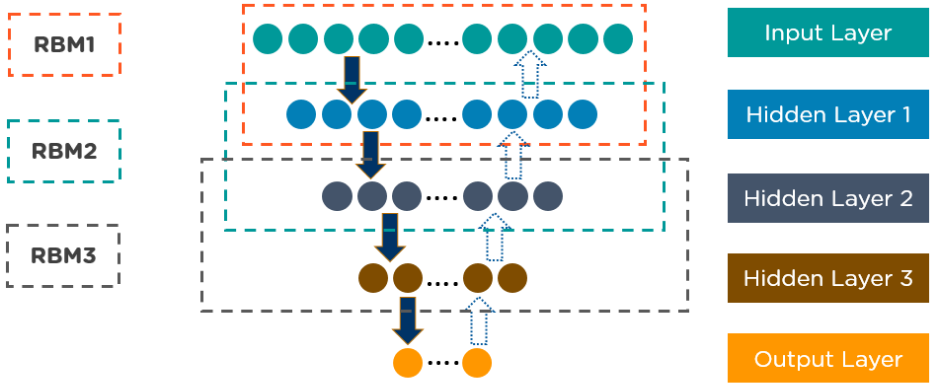### 9. Restricted Boltzmann Machines (RBMs)

Developed by Geoffrey Hinton, RBMs are stochastic neural networks that can learn from a probability distribution over a set of inputs.

This deep learning algorithm is used for dimensionality reduction, classification, regression, collaborative filtering, feature learning, and topic modeling. RBMs constitute the building blocks of DBNs.

RBMs consist of two layers:

• Visible units
• Hidden units

Each visible unit is connected to all hidden units. RBMs have a bias unit that is connected to all the visible units and the hidden units, and they have no output nodes.

#### How Do RBMs Work?

RBMs have two phases: forward pass and backward pass.

• RBMs accept the inputs and translate them into a set of numbers that encodes the inputs in the forward pass.
• RBMs combine every input with individual weight and one overall bias. The algorithm passes the output to the hidden layer.
• In the backward pass, RBMs take that set of numbers and translate them to form the reconstructed inputs.
• RBMs combine each activation with individual weight and overall bias and pass the output to the visible layer for reconstruction.
• At the visible layer, the RBM compares the reconstruction with the original input to analyze the quality of the result.

Below is a diagram of how RBMs function: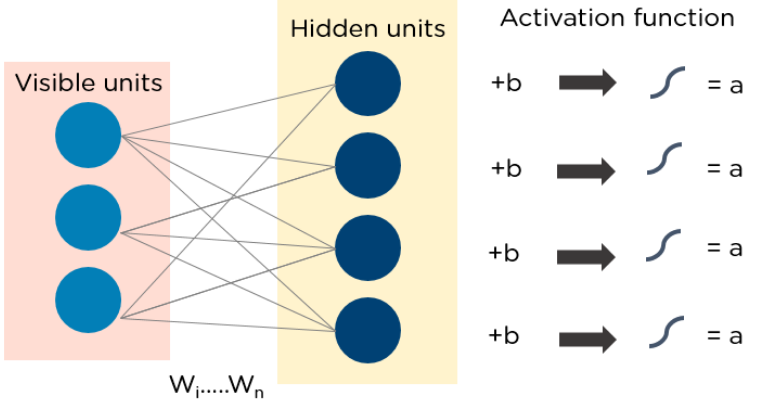### 10. Autoencoders

Autoencoders are a specific type of feedforward neural network in which the input and output are identical. Geoffrey Hinton designed autoencoders in the 1980s to solve unsupervised learning problems. They are trained neural networks that replicate the data from the input layer to the output layer. Autoencoders are used for purposes such as pharmaceutical discovery, popularity prediction, and image processing.

#### How Do Autoencoders Work?

An autoencoder consists of three main components: the encoder, the code, and the decoder.

• Autoencoders are structured to receive an input and transform it into a different representation. They then attempt to reconstruct the original input as accurately as possible.
• When an image of a digit is not clearly visible, it feeds to an autoencoder neural network.
• Autoencoders first encode the image, then reduce the size of the input into a smaller representation.
• Finally, the autoencoder decodes the image to generate the reconstructed image.

The following image demonstrates how autoencoders operate: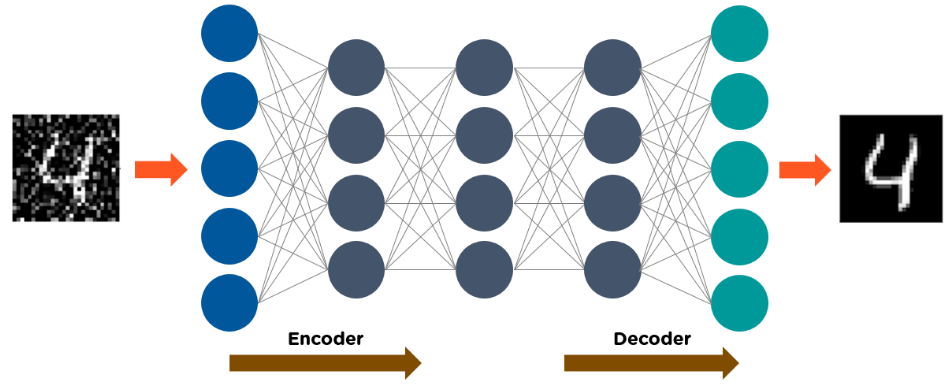This article has been published from the source link without modifications to the text. Only the headline has been changed.

Source link

- Advertisment -### Creating and Deploying an Ethereum Smart Contract

- Advertisment -